# Texas Go Math Grade 1 Lesson 5.1 Answer Key Use Pictures to Show Taking From

Refer to our Texas Go Math Grade 1 Answer Key Pdf to score good marks in the exams. Test yourself by practicing the problems from Texas Go Math Grade 1 Lesson 5.1 Answer Key Use Pictures to Show Taking From.

## Texas Go Math Grade 1 Lesson 5.1 Answer Key Use Pictures to Show Taking From

Essential Question
How can you show taking from, or separating, with pictures?
Explanation:
Taking from are separating the picture says the subtraction.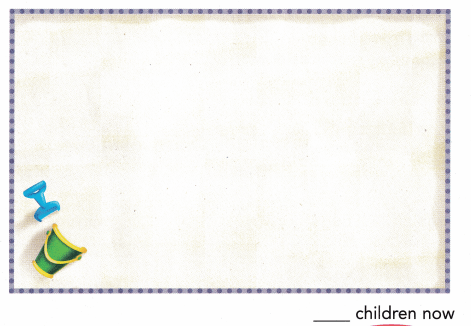For The Teacher

• Read the following problem. Have children draw a picture to show the problem. There are 5 children in the sandbox. 2 walk away. How many children are in the sandbox now?
Explanation: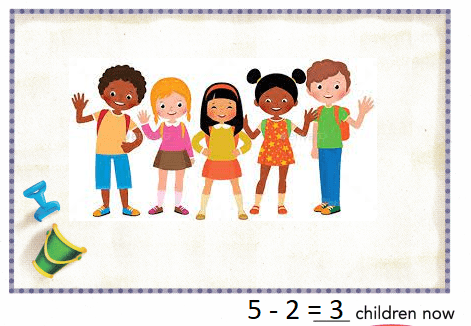5 – 2 = 3
3 children are in the sandbox now

Math Talk
Mathematical processes
How did you find how many are in the sandbox now? Explain.
Explanation:
5 – 2 = 3
By subtracting 2 from the 5 we got 3

Model and Draw

There are 4 cats in the whole group.3 cats now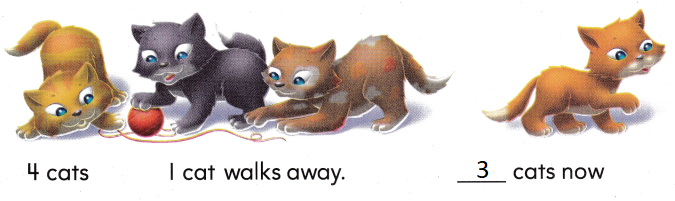Explanation:
4 – 1 =3

Share and Show

Circle the part you are taking from, or separating from, the group. Then cross it out. Write how many there are now.

Question 1.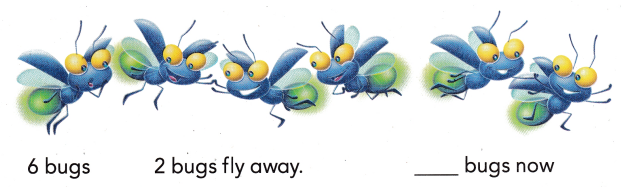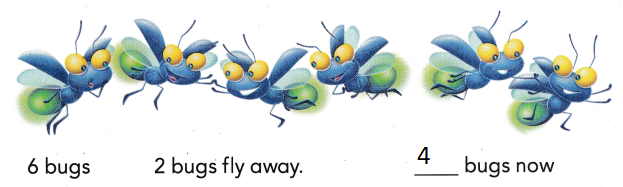Explanation:
6 – 2 = 4

Question 2.There are 3 dogs. 1 dog walks away. How many dogs are there now?
____ dogs now
Explanation:
3 – 1 = 2

Problem Solving

Circle the part you are taking from, or separating, from the group. Then cross it out. Write how many there are now.

Question 3.There are 7 chicks.
2 chicks walk away.
How many chicks are there now?
___ chicks now
Explanation:
7 – 2 = 5

Write or draw to solve.

Question 4.
There are 6 cats. 1 cat runs away. How many cats are there now?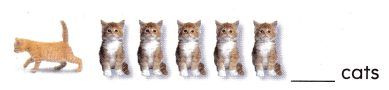Explanation:
7 – 1 = 6

Question 5.
Ellie and Sara see 8 birds in the tree. Elije sees 2 fewer birds than Sara. Sara sees 5 birds. How many birds does Elije see?Explanation:
Elije sees 2 fewer birds than Sara.
Sara sees 5 birds. So Elije sees sees 5- 2 = 3 .

Question 6.
Multi-Step Choose numbers to complete the story. Write the numbers. Draw to show the problem.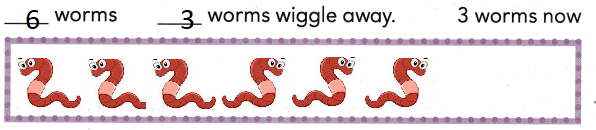Explanation:
there are 6 worms
3 wiggle away
6 – 3 = 3
so, 3 remains.

Question 7.
There are 7 fish. 3 fish swim away. How many fish are there now?
(A) 4
(B) 10
(C) 6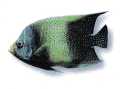Explanation:
7 – 3 = 4

Question 8.
There are 8 turtles. 3 turtles walk away. How many turtles are there now?(A) 4
(B) 3
(C) 5
Explanation:
8- 3 = 5

Question 9.
Use Tools 9 birds are in a tree. 3 birds fly away. Then 2 more birds fly away. How many birds are in the tree now?
(A) 3
(B) 4
(C) 6Explanation:
9 birds are in a tree. 3 birds fly away
9 – 3 = 6
Then 2 more birds fly away
6 – 2 = 4

Question 10.
Texas Test Prep There are 7 whales. 2 whales swim away. How many whales are there now?Take Home Activity

• Have your child draw and solve the subtraction problem: There are 6 cows, 3 cows walk away. How many cows are there now?

### Texas Go Math Grade 1 Lesson 5.1 Homework and Practice Answer Key

Circle the part you are taking from, or separating from, the group. Then cross it out. Write how many there are now.

Question 1.Explanation:
8 – 2 = 6

Question 2.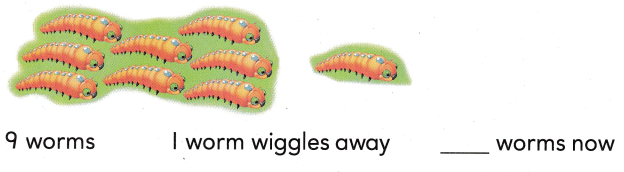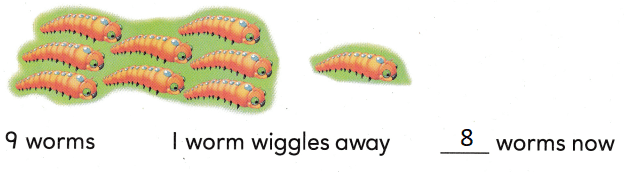Explanation:
9 – 1 = 8

Problem Solving

Choose numbers to complete the story. Write the numbers.

Question 3.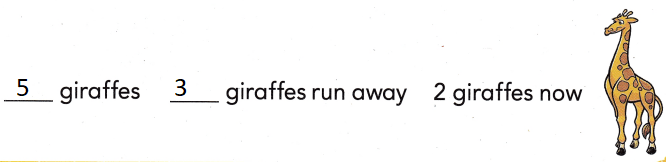Explanation:
5 – 3 = 2

Texas Test Prep

Question 4.
There are 6 rabbits. 3 rabbits hop away. Hõw many rabbits are there now?
(A) 3
(B) 9
(C) 6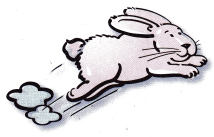Explanation:
6 – 3 = 3
Question 5.
8 ants are in the grass. 3 ants walk away. How many ants are there now?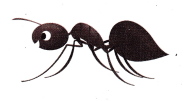(A) 11
(B) 8
(C) 4
8 – 3 = 5
Explanation:
8 – 3 = 5
5 ants are there now

Question 6.
Multi-Step Carl sees 7 dogs. All sees 3 fewer dogs than Carl. Tim sees 1 fewer dog than All. How many dogs does Tim see?
(A) 6
(B) 3
(C) 4
Explanation:
Carl sees 7 dogs. All sees 3 fewer dogs than Carl. then all see
7 – 3 = 4
Tim sees 1 fewer dog than All then Tim see
4 – 1 = 3

Question 7.
There are 8 snakes. 2 snakes crawl away. How many snakes are there now?
(A) 10
(B) 8
(C) 6